Test: Polynomials - 2

# Test: Polynomials - 2

Test Description

## 25 Questions MCQ Test Mathematics (Maths) Class 9 | Test: Polynomials - 2

Test: Polynomials - 2 for Class 9 2023 is part of Mathematics (Maths) Class 9 preparation. The Test: Polynomials - 2 questions and answers have been prepared according to the Class 9 exam syllabus.The Test: Polynomials - 2 MCQs are made for Class 9 2023 Exam. Find important definitions, questions, notes, meanings, examples, exercises, MCQs and online tests for Test: Polynomials - 2 below.
Solutions of Test: Polynomials - 2 questions in English are available as part of our Mathematics (Maths) Class 9 for Class 9 & Test: Polynomials - 2 solutions in Hindi for Mathematics (Maths) Class 9 course. Download more important topics, notes, lectures and mock test series for Class 9 Exam by signing up for free. Attempt Test: Polynomials - 2 | 25 questions in 25 minutes | Mock test for Class 9 preparation | Free important questions MCQ to study Mathematics (Maths) Class 9 for Class 9 Exam | Download free PDF with solutions
 1 Crore+ students have signed up on EduRev. Have you?
Test: Polynomials - 2 - Question 1

### √2 is a polynomial of degree

Test: Polynomials - 2 - Question 2

### If a+b+c = 0, then a3+b3+c3 is equal to

Test: Polynomials - 2 - Question 3

### A polynomial containing two nonzero terms is called a ________.

Detailed Solution for Test: Polynomials - 2 - Question 3
A binomial is a mathematical expression with two terms.
Examples of binomials.
binomial
All of these examples are binomials. Study them for a bit, and see if you can spot a pattern. The following is a list of what binomials must have:
They must have two terms.
If the variables are the same, then the exponents must be different.
Exponents must be whole positive integers. They cannot be negatives or fractions.
A term is a combination of numbers and variables. In the example 3x + 5, our first term is 3x, and our second term is 5. Terms are separated by either addition or subtraction. In our first example, notice how the 3x and 5 are separated by addition. In the last example, we have a binomial whose two terms both have the same variable s. Notice how each term has its variable to a different exponent. The first term has an exponent of 5, and the second term has an exponent of 4. While we can have fractions for our numbers, we cannot have fractional exponents.
Here are some examples of expressions that are not binomials.
Test: Polynomials - 2 - Question 4

The expanded form of (3x−5)3 is

Detailed Solution for Test: Polynomials - 2 - Question 4

Let a = 3x and b = 5 (3x)³ - (5)³ - 3×3x×5(3x- 5) = 27x³ - 125 -45x ( 3x -5) = 27x³ -125 - 135x² + 225x = 27x³ - 135x² + 225x - 125

Test: Polynomials - 2 - Question 5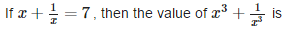Detailed Solution for Test: Polynomials - 2 - Question 5

X+ 1/x = 7 then, cubing both side = (x +1/x)³ = (7)³ = x³ + 1/x³ + 3×X×1/X (x+1/x) = 343 = x³ +1/x³ +3(7) = 343 =x³ +1/x³ +21 =343 = x³ +1/x³ =343 - 21 = x³ +1/x³ = 322

Test: Polynomials - 2 - Question 6

Degree of the polynomial 4x4 + 0x3 + 0x5 + 5x + 7 is:

Detailed Solution for Test: Polynomials - 2 - Question 6

Degree is the highest exponent of any value in an equation. Here, this highest exponent is 4. Therefore, 4 is the degree of the given equation.

Test: Polynomials - 2 - Question 7

(x + 1) is a factor of the polynomial

Test: Polynomials - 2 - Question 8

A polynomial containing three nonzero terms is called a ________.

Test: Polynomials - 2 - Question 9

If x + 2 is a factor of x3 – 2ax2 + 16, then value of a is

Detailed Solution for Test: Polynomials - 2 - Question 9

use factor theorem as x+2 is factor of
x³-2ax²+16 so put x = -2 and equate the equation to 0
so putting x = -2
(-2)³-2a(-2)²+16 =0
-8-8a+16=0
-8a = -8
a = 8/8= 1
So,
a = 1

Test: Polynomials - 2 - Question 10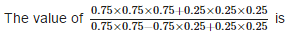Detailed Solution for Test: Polynomials - 2 - Question 10

Solution :- (0.75 * 0.75 * 0.75 + 0.25 * 0.25 * 0.25)/(0.75 * 0.75 - 0.75 * 0.25 + 0.25 * 0.25)

= (0.421875 + 0.015625)/(0.5625 - 0.1875 + 0.0625)

= (0.4375)/(0.4375)

= 1

Test: Polynomials - 2 - Question 11

If one of the factor of x2 + x – 20 is (x + 5). Find the other

Test: Polynomials - 2 - Question 12

If 10x−4x2−3, then the value of p(0)+p(1) is

Test: Polynomials - 2 - Question 13

The coefficient of x3 in 2x+x2−5x3+x4 is

Test: Polynomials - 2 - Question 14

If both x - 2 andare the factors of px2 + 5x + r, then

Detailed Solution for Test: Polynomials - 2 - Question 14

Let f(x) = px2 + 5 x + r
If (x - 2) is a factor of f (x), then by factor theorem
f(2) = 0 | x - 2 = 0 ⇒ x = 2
⇒ p(2)2 + 5(2) + r = 0
⇒ 4p + r + 10 = 0    ...(1)

Ifis a factor of f (x), then by factor theorem,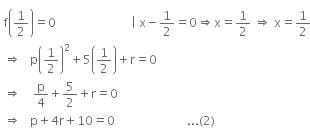Subtracting (2) from (1), we get
3p - 3r = 0
⇒    p = r

Test: Polynomials - 2 - Question 15

If x + 2 is a factor of x3 – 2ax2 + 16, then value of a is

Test: Polynomials - 2 - Question 16

The value of the polynomial 5x−4x2+35, when x = −1 is

Test: Polynomials - 2 - Question 17

If x2+kx+6 = (x+2)(x+3), then the value of ‘k’ is

Test: Polynomials - 2 - Question 18

The remainder when the polynomial x4+2x3−3x2+x−1 is divided by (x−2) is

Test: Polynomials - 2 - Question 19

The remainder obtained when the polynomial p(x) is divided by (b – ax) is

Detailed Solution for Test: Polynomials - 2 - Question 19

Solution :- b-ax=0

b=ax

b/a=x

i.e. remainder is p(b/a)

Test: Polynomials - 2 - Question 20

The value of x3+y3+15xy−125 when x+y = 5 is

Detailed Solution for Test: Polynomials - 2 - Question 20

x³+y³+15xy-125
=x³ + y³ +3 xy ×5 - 125
=x³ + y³ +3xy(x+y) - (5)³
= (x+y) ³ - (5)³
=(5)³ - (5)³
=0

Test: Polynomials - 2 - Question 21

If p(x) = x + 3, then p(x) + p(-x) is equal to

Detailed Solution for Test: Polynomials - 2 - Question 21
Given p(x) = x+3, put x = -x in the given equation, we get p(-x) = -x+3
Now, p(x)+ p(-x) = x+ 3+ (-x)+ 3=6
Test: Polynomials - 2 - Question 22

One of the factors of (16y2−1)+(1−4y)2 is

Detailed Solution for Test: Polynomials - 2 - Question 22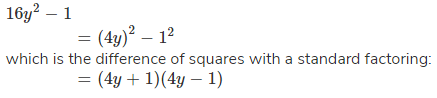Test: Polynomials - 2 - Question 23

If the polynomial x3−6x2+ax+3 leaves a remainder 7 when divided by (x−1), then the value of ‘a’ is

Test: Polynomials - 2 - Question 24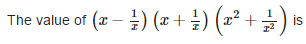Test: Polynomials - 2 - Question 25

The value of (a2−b2)3+(b2−c2)3+(c2−a2)3 is

Detailed Solution for Test: Polynomials - 2 - Question 25 Let (a²-b²) =x , (b²-c²) =y , (c²-a²) = z we know that a+b+ c=0 and, a³ + b³ + c³ = 3abc so, 3(a+b)(a-b)(b+c) (b-c)(c+a)(c-a) = 3(a+b)(b+c)(c+a)(a-b)(b-c)(c-a)

## Mathematics (Maths) Class 9

88 videos|397 docs|109 tests
 Use Code STAYHOME200 and get INR 200 additional OFF Use Coupon Code
Information about Test: Polynomials - 2 Page
In this test you can find the Exam questions for Test: Polynomials - 2 solved & explained in the simplest way possible. Besides giving Questions and answers for Test: Polynomials - 2, EduRev gives you an ample number of Online tests for practice

## Mathematics (Maths) Class 9

88 videos|397 docs|109 tests